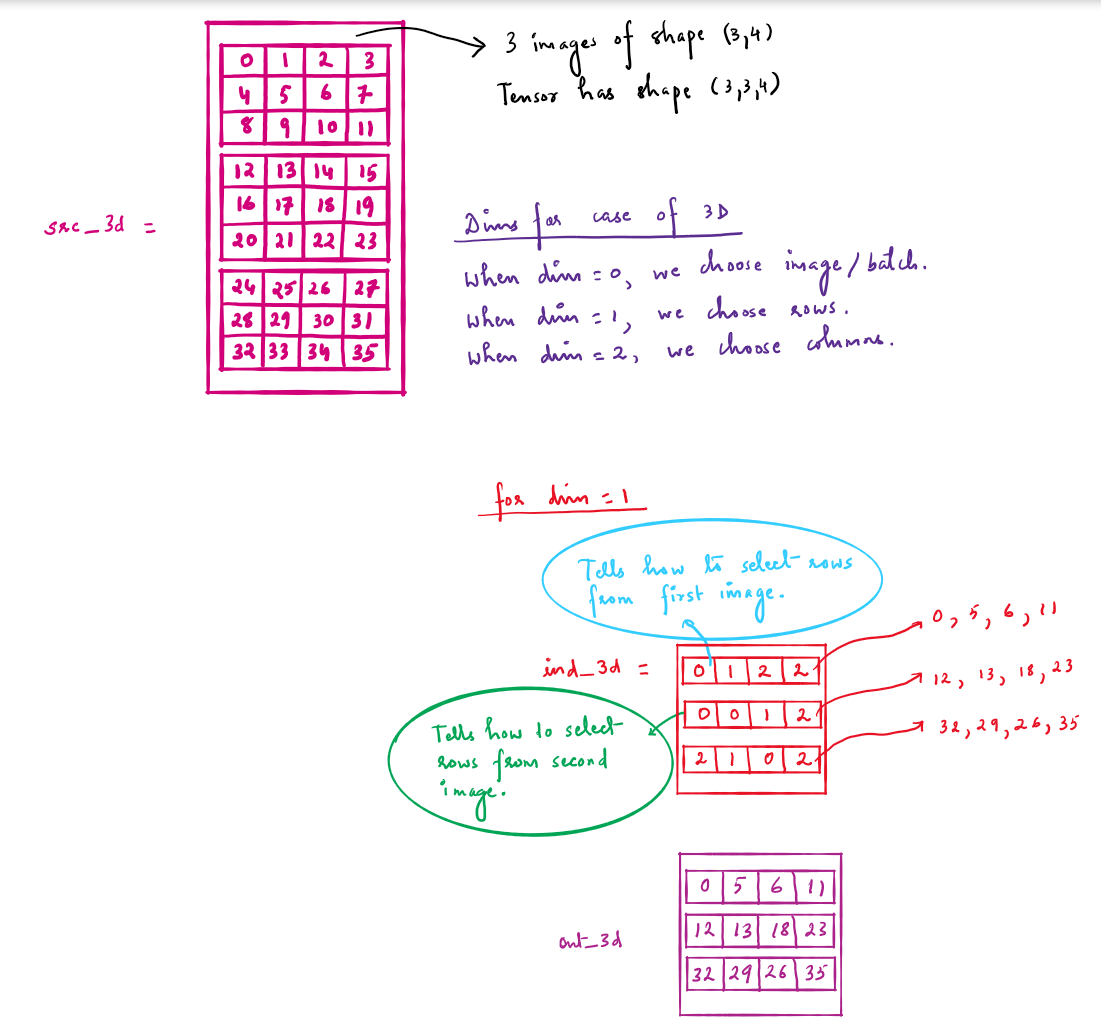# Understanding torch.gather function in Pytorch

Original article was published by Pranav Chaturvedi on Deep Learning on Medium# Understanding torch.gather function in Pytorch

Two arguments of this function, index and dim are the key to understanding this function.

For case of 2D, dim = 0 corresponds to rows and dim = 1 corresponds to columns.

For case of 3D, dim = 0 corresponds to image or batch, dim = 1 corresponds to rows and dim = 2 corresponds to columns.

# Case of 2D input tensor

## 1. Understanding dim argument:

a. When dim = 0, we choose rows.

b. When dim = 1, we choose columns.

## 2. Understanding index argument:

a. Index argument will have same no of dimensions as input(does not mean shape will be same).

b. Output tensor will have the same shape as index tensor.

c. The elements of index tensor tell which row (for dim = 0, 2D case) to choose and position of the particular element tells which column to choose.

d. The elements of index tensor tell which column (for dim = 1, 2D case) to choose and position of the particular element tells which row to choose.

# Case of 3D input tensor

## 1. Understanding dim argument:

a. When dim = 0, we choose image or batch.

b. When dim = 1, we choose rows.

c. When dim = 2, we choose columns.

## 2. Understanding index argument:

a. a and b from above, Case of 2D input tensor apply.

b. The elements of index tensor tell which image or batch (for dim = 0, 3D case) to choose and position of the particular element tells which rows and columns to choose and so on for dim = 1 and dim = 2.

Let’s take two examples for case of 2D.

1st Example

When dim = 0, and

ind_2d = [[3, 2, 0, 1]]

ind_2d has shape (1, 4) so output will have same shape.

0th element of ind_2d, i.e. 3 tells we choose 3rd row and 0th column (since 3 is 0th element of index tensor).

1st element of ind_2d i.e. 2 tells we choose 2nd row starting from 0 (row because dim = 0) and 1st column (since 1st element of index tensor). And so on.

2nd Example

Let’s suppose from above tensor we want to select 0, 6, 10 and 15. (Tip: We read these number order-wise from up to down, so we form a column like index tensor. When we read from left to right, we form row like index tensor.)

Now 0 belongs to 0th column, 6 belongs to 2nd column, 10 belongs to 2nd column and 15 belongs to 3rd column.

So our index tensor is [[0, 2, 2, 3]] in column form i.e. of shape (4, 1). And since we’ve selected columns, therefore dim = 1.

There’s no way we could have selected rows and got the desired output tensor.

## The case of 3D is very similar. Get the ideas from infographic below.

Here is the link to a python notebook with several examples for both cases of 2D and 3D input tensor:

Link to the Pytorch function :

https://pytorch.org/docs/stable/generated/torch.gather.html?highlight=torch%20gather#torch.gather